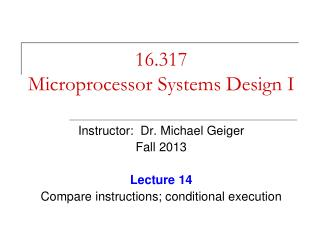DownloadDownload Presentation16.317 Microprocessor Systems Design I

# 16.317 Microprocessor Systems Design I

Download Presentation## 16.317 Microprocessor Systems Design I

- - - - - - - - - - - - - - - - - - - - - - - - - - - E N D - - - - - - - - - - - - - - - - - - - - - - - - - - -
##### Presentation Transcript

1. 16.317Microprocessor Systems Design I Instructor: Dr. Michael Geiger Fall 2013 Lecture 14 Compare instructions; conditional execution

2. Lecture outline • Announcements/reminders • HW 4 to be posted; due 10/16 • Exam 1 regrade requests due 10/11 • Today’s lecture • Compare instructions • CMOV • Set on condition • Early feedback forms Microprocessors I: Lecture 14

3. Compare Instructions • Compare 2 values; store result in ZF/SF • General format: CMP D,S • Works by performing subtraction (D) – (S) • D, S unchanged • ZF/SF/OF indicate result (signed values) • ZF = 1  D == S • ZF = 0, (SF XOR OF) = 1  D < S • ZF = 0, (SF XOR OF) = 0  D > S Microprocessors I: Lecture 14

4. Example—Initialization of internal registers with immediate data and compare. Example: MOV AX,1234H ;Initialize AX MOV BX,ABCDH ;Initialize BX CMP AX,BX ;Compare AX-BX Data registers AX and BX initialized from immediate data IMM16  (AX) = 1234H  + integer IMM16  (BX) = ABCDH  - integer Compare computation performed as: (AX) = 00010010001101002 (BX) = 10101011110011012 (AX) – (BX) = 00010010001101002 - 10101011110011012 ZF = 0 = NZ SF = 0 = PL ;treats as signed numbers CF = 1 = CY AF = 1 = AC OF = 0 = NV PF = 0 = PO Compare Instructions- Example Microprocessors I: Lecture 14

5. Condition codes • Conditional execution: result depends on value of flag bit(s) • Intel instructions specify condition codes • Condition code implies certain flag values • Opcodes written with cc as part of name • cc can be replaced by any valid code • Examples: CMOVcc, SETcc, Jcc • Specific examples: CMOVE, SETL, SETZ, JNE, JG Microprocessors I: Lecture 14

6. Condition codes (cont.) • Testing overflow • O (OF = 1), NO (OF =0) • Testing carry flag • C (CF = 1) • NC (CF = 0) • Testing sign flag • S (SF = 1), NS (SF = 0) • Testing parity flag • P or PE (PF = 1) • NP or PO (PF = 0) Microprocessors I: Lecture 14

7. Condition codes (cont.) • Testing equality/zero result • E or Z (ZF = 1) • NE or NZ (ZF = 0) • Signed comparison • L or NGE (SF XOR OF = 1) • NL or GE (SF XOR OF = 0) • LE or NG ((SF XOR OF) OR ZF = 1) • NLE or G ((SF XOR OF) OR ZF = 0) • Unsigned comparison • “Below”  less than,“above”  greater than • B, NAE (CF = 1) • NB, AE (CF = 0) • BE or NA (CF OR ZF = 1) • NBE or A (CF OR ZF = 0) Microprocessors I: Lecture 14

8. Conditional move (CMOV) • Only in Pentium Pro & later • Perform move only if condition is true • Examples: • CMOVZ AX, [SI]  move if ZF == 1 • CMOVG EBX, EAX  move if greater than Microprocessors I: Lecture 14

9. Byte Set on Condition Instruction • Byte set on condition instruction • Used to set byte based on condition code • Can be used for boolean results—complex conditions • General format: • SETcc D • cc = one of the supported conditional relationships Microprocessors I: Lecture 14

10. Byte Set on Condition Instruction • Operation: Flags tested for conditions defined by “cc” and the destination in a register or memory updated as follows If cc test True: 000000012 = 01H  D If cc test False: 000000002 = 00H  D • Examples of conditional tests: SETE = set byte if equal  ZF = 1 SETC = set byte if carry  CF =1 SETBE = set byte if below or equal  CF = 1 +(or) ZF = 1 • Example: SETA AL = set byte if above if CF = 0  (and) ZF = 0 (AL) = 01H Otherwise, (AL) =00H Microprocessors I: Lecture 14

11. Example • Show the results of the following instructions, assuming that • “A” = DS:100H = 0001H • “B” = DS:102H = 0003H • “C” = DS:104H = 1011H • “D” = DS:106H = 1011H • “E” = DS:108H = ABCDH • “F” = DS:10AH = DCBAH • What complex condition does this sequence test? • MOV AX, [100H] • CMP AX, [102H] • SETLE BL • MOV AX, [104H] • CMP AX, [106H] • SETE BH • AND BL, BH • MOV AX, [108H] • CMP AX, [10AH] • SETNE BH • OR BL, BH Microprocessors I: Lecture 14

12. Example solution • Condition being tested: • To simplify, treat each word as a variable named “A” through “F” • ((A <= B) && (C == D)) || (E != F) • Source: http://www.arl.wustl.edu/~lockwood/class/cs306/books/artofasm/Chapter_6/CH06-4.html Microprocessors I: Lecture 14

13. Final notes • Next time: • Jump and loop instructions • Reminders: • HW 4 to be posted; due 10/16 • Exam 1 regrade requests due 10/11 Microprocessors I: Lecture 14